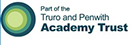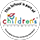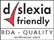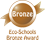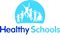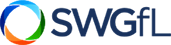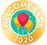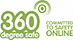# Calculation Policy

Introduction

This policy aims to develop, model and explain core understandings and mathematical principles and progression to ensure consistency in the teaching and learning of mathematics in our schools.

The focus of this policy is the calculation of the four mathematical operations with an emphasis on written strategies to clarify processes and understanding and to make direct links to mental calculating.  It is crucial that these mental strategies are discretely taught and linked to written strategies and not confined to starter activities in lessons.

The overall aims of this policy are that, when children leave primary school they:

• have a secure knowledge of number facts and a good understanding of the four operations supported by a fluency and understanding of the fundamentals of mathematics

• includes the idea of variation theory which is linked to mastery and has been adapted following the joint England Shanghai maths project of 2014/15 which the school was a part of.  This is taking known facts and repeating these to support the application of skills.

• know the best strategy to use, estimate before calculating, systematically break problems down into a series of simpler steps with perseverance and use estimation and rounding to check that an answer is reasonable

• are able to use this knowledge and understanding to carry out calculations mentally, solve problems of increasing complexity and develop an ability to recall and apply knowledge rapidly.

• make use of diagrams and informal notes and jottings to help record steps and partial answers when using mental methods

• have an efficient, reliable, compact written method of calculation for each operation, which they can apply with confidence when undertaking calculations

• be able to identify when a calculator is the best tool for the task and use this primarily as a way of checking rather than simply a way of calculating.

• be able to explain their strategies to calculate and, using spoken language, give mathematical justification, argument or proof.